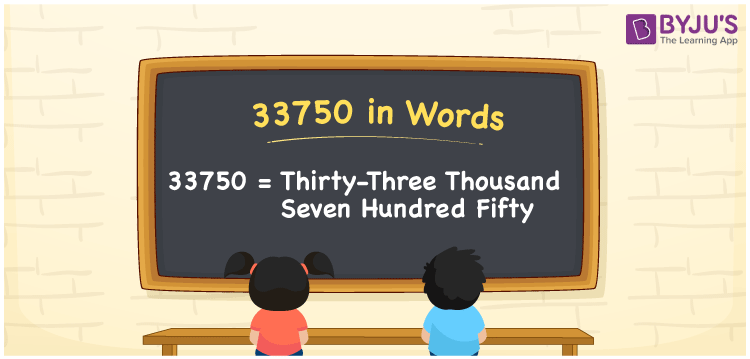# 33750 in words

33750 in words is written as Thirty Three Thousand Seven Hundred and Fifty. In 33750, the first 3 has a place value of ten thousand, second 3 is in the place value of thousand, 7 is in the place value of hundred and 5 is in the place value of ten. The article on Place Value gives more information. The number 33750 is used in expressions that relate to money, distance, social media views, and many more. For example, “The video had Thirty Three Thousand Seven Hundred and Fifty views only.”

 33750 in words Thirty Three Thousand Seven Hundred and Fifty Thirty Three Thousand Seven Hundred and Fifty in Numbers 33750

## 33750 in English Words## How to Write 33750 in Words?

We can convert 33750 to words using a place value chart. The number 33750 has 5 digits, so let’s make a chart that shows the place value up to 5 digits.

 Ten thousand Thousands Hundreds Tens Ones 3 3 7 5 0

Thus, we can write the expanded form as:

3 × Ten thousand + 3 × Thousand + 7 × Hundred + 5 × Ten + 0 × One

= 3 × 10000 + 3 × 1000 + 7 × 100 + 5 × 10 + 0 × 1

= 33750.

= Thirty Three Thousand Seven Hundred and Fifty.

33750 is the natural number that is succeeded by 33749 and preceded by 33751.

33750 in words – Thirty Three Thousand Seven Hundred and Fifty.

Is 33750 an odd number? – No.

Is 33750 an even number? – Yes.

Is 33750 a perfect square number? – No.

Is 33750 a perfect cube number? – No.

Is 33750 a prime number? – No.

Is 33750 a composite number? – Yes.

## Solved Example

1. Write the number 33750 in expanded form

Solution: 3 x 10000 + 3 x 1000 + 7 x 100 + 5 x 10 + 0 x 1

Or Just 3 x 10000 + 3 x 1000 + 7 x 100 + 5 x 10

We can write 33750 = 30000 + 3000 + 700 + 50 + 0

= 3 x 10000 + 3 x 1000 + 7 x 100 + 5 x 10 + 0 x 1.

## Frequently Asked Questions on 33750 in words

Q1

### How to write the number 33750 in words?

33750 in words is written as Thirty Three Thousand Seven Hundred and Fifty.
Q2

### State whether True or False. 33750 is divisible by 3?

True. 33750 is divisible by 3.
Q3

### Is 33750 divisible by 10?

Yes. 33750 is divisible by 10.